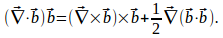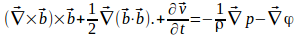## Equation if Motion For an Inviscid, Incompressible Fluid

For an incompressible fluid,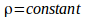so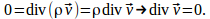If the body force per unit volume is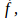applying Newton's Second Law to a cube of unit volume to obtain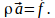We can also write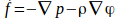where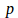is the pressure in the fluid.

Equating these gives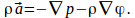(1)

Consider the motion of a particle in the fluid from position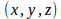to position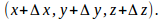Let the velocity atbe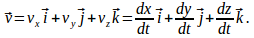The velocity at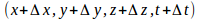will be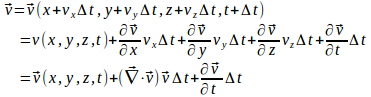Then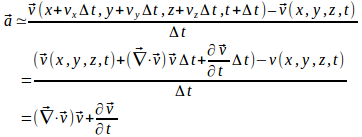Substitute this into (1) to obtain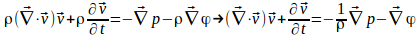We can define a vector field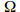called the vorticity as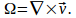Use the identity